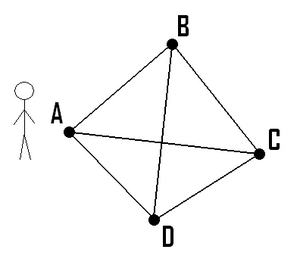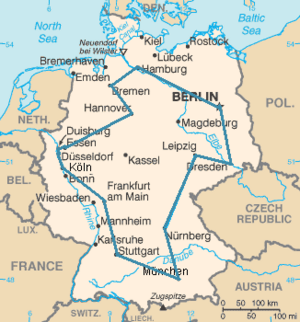# Travelling salesman problem facts for kids

Kids Encyclopedia Facts
(Redirected from Travelling Salesman Problem)S salesman wants to visit all cities,A, B, C and D. What is the best way to do this (cheapest airline tickets, and minimal travel time)?Optimal route of a salesman visiting the 15 biggest cities in Germany. The route shown is the shortest of the 43,589,145,600 possible ones.

The Travelling Salesman Problem (often called TSP) is a classic algorithmic problem in the field of computer science. It is focused on optimization. In this context better solution often means a solution that is cheaper. TSP is a mathematical problem. It is most easily expressed as a graph describing the locations of a set of nodes.

The travelling salesman problem was defined in the 1800s by the Irish mathematician W. R. Hamilton and by the British mathematician Thomas Kirkman. Hamilton’s Icosian Game was a recreational puzzle based on finding a Hamiltonian cycle. The general form of the TSP appears to have been first studied by mathematicians during the 1930s in Vienna and at Harvard, notably by Karl Menger. Menger defines the problem, considers the obvious brute-force algorithm, and observes the non-optimality of the nearest neighbour heuristic:

We denote by messenger problem (since in practice this question should be solved by each postman, anyway also by many travelers) the task to find, for ﬁnitely many points whose pairwise distances are known, the shortest route connecting the points. Of course, this problem is solvable by finitely many trials. Rules which would push the number of trials below the number of permutations of the given points, are not known. The rule that one first should go from the starting point to the closest point, then to the point closest to this, etc., in general does not yield the shortest route.

Hassler Whitney at Princeton University introduced the name travelling salesman problem soon after.

## Stating the problem

The Travelling Salesman Problem describes a salesman who must travel between N cities. The order in which he does so is something he does not care about, as long as he visits each one during his trip, and finishes where he was at first. Each city is connected to other close by cities, or nodes, by airplanes, or by road or railway. Each of those links between the cities has one or more weights (or the cost) attached. The cost describes how "difficult" it is to traverse this edge on the graph, and may be given, for example, by the cost of an airplane ticket or train ticket, or perhaps by the length of the edge, or time required to complete the traversal. The salesman wants to keep both the travel costs, as well as the distance he travels as low as possible.

The Traveling Salesman Problem is typical of a large class of "hard" optimization problems that have intrigued mathematicians and computer scientists for years. Most important, it has applications in science and engineering. For example, in the manufacture of a circuit board, it is important to determine the best order in which a laser will drill thousands of holes. An efficient solution to this problem reduces production costs for the manufacturer.

## Difficulty

The travelling salesman problem is regarded as difficult to solve. If there is a way to break this problem into smaller component problems, the components will be at least as complex as the original one. This is what computer scientists call NP-hard problems.

Many people have studied this problem. The easiest (and most expensive solution) is to simply try all possibilities. The problem with this is that for N cities you have (N-1) factorial possibilities. This means that for only 10 cities there are over 180 thousand combinations to try (since the start city is defined, there can be permutations on the remaining nine). We only count half since each route has an equal route in reverse with the same length or cost. 9! / 2 = 181 440

• Exact solutions to the problem can be found, using branch and bound algorithms. This is currently possible for up to 85,900 cities.
• Heuristics approaches use a set of guiding rules for selection of the next node. But since heuristics result in approximations, they will not always give the optimal solution, although high quality admissible heuristics can find a useful solution in a fraction of the time required for a full brute force of the problem. An example of a heuristic for a node would be a summation of how many unvisited nodes are "close by" a connected node. This could encourage the salesman to visit a group of close-by nodes clustered together before moving onto another natural cluster in the graph. See Monte Carlo algorithms and Las Vegas algorithms

## Images for kidsTravelling salesman problem Facts for Kids. Kiddle Encyclopedia.